# 后 CNN 探索，如何用 RNN 进行图像分类

• 2022-10-13
中国香港
• 本文字数：3880 字

阅读完需：约 13 分钟## 一、 写前的思考：

CNN 和 RNN 直接的不同点；

CNN 进行空间扩展，神经元与特征卷积；RNN 进行时间扩展，神经元与多个时间输出计算；

RNN 可以用于描述时间上连续状态的输出，有记忆功能；CNN 则用于静态输出；

CNN 高级结构可以达到 100+深度；RNN 的深度有限。

CNN 和 RNN 组合的意义：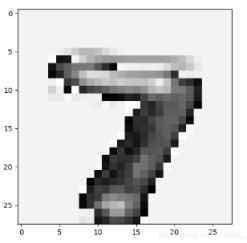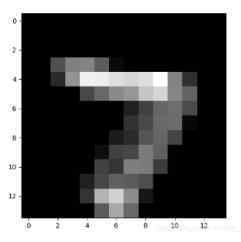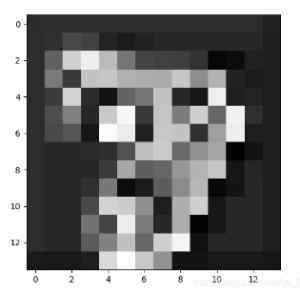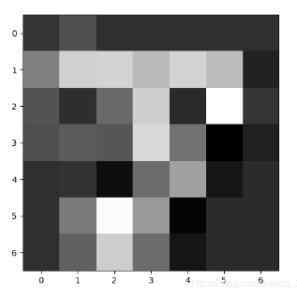## 二、 如何将图像数据改成序列数据？如何加入 RNN 系列程序，以改进图片分类的性能？

outputs, states = tf.nn.dynamic_rnn(rnn_cell, X, dtype=tf.float32)即可将 X 应用于 RNN 程序

import matplotlib.pyplot as plt # plt 用于显示图片from PIL import Imageimport numpy as npimage1 = Image.open('./1.jpg')img=np.array(image1)# 通道转换def change_image_channels(image):# 3通道转单通道if image.mode == 'RGB':r, g, b = image.split()return r,g,bima = change_image_channels(image1)for i in range(3):plt.imshow(ima[i])plt.show()r=img[:,:,0]g=img[:,:,1]b=img[:,:,2]GRAY = b * 0.114 + g * 0.387 + r * 0.29im=Image.fromarray(GRAY) # numpy 转 image类im.show()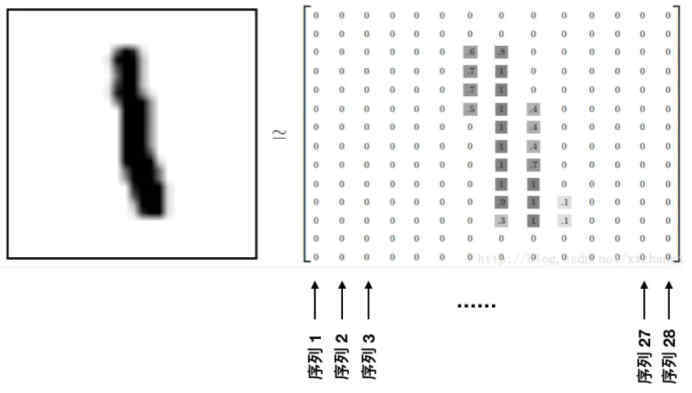import syssys.path.append('..')import torchfrom torch.autograd import Variablefrom torch import nnfrom torch.utils.data import DataLoaderfrom torchvision import transforms as tfsfrom torchvision.datasets import MNIST# 定义数据data_tf = tfs.Compose([    tfs.ToTensor(),    tfs.Normalize([0.5], [0.5]) # 标准化])train_set = MNIST('./data', train=True, transform=data_tf, download=True)test_set = MNIST('./data', train=False, transform=data_tf, download=True)train_data = DataLoader(train_set, 64, True, num_workers=2)test_data = DataLoader(test_set, 128, False, num_workers=2)# 定义模型class rnn_classify(nn.Module):    def __init__(self, in_feature=28, hidden_feature=100, num_class=10, num_layers=2):        super(rnn_classify, self).__init__()        self.rnn = nn.LSTM(in_feature, hidden_feature, num_layers) # 使用两层 lstm        self.classifier = nn.Linear(hidden_feature, num_class) # 将最后一个 rnn 的输出使用全连接得到最后的分类结果    def forward(self, x):        '''        x 大小为 (batch, 1, 28, 28)，所以我们需要将其转换成 RNN 的输入形式，即 (28, batch, 28)        '''        x = x.squeeze() # 去掉 (batch, 1, 28, 28) 中的 1，变成 (batch, 28, 28)        x = x.permute(2, 0, 1) # 将最后一维放到第一维，变成 (28, batch, 28)        out, _ = self.rnn(x) # 使用默认的隐藏状态，得到的 out 是 (28, batch, hidden_feature)        out = out[-1, :, :] # 取序列中的最后一个，大小是 (batch, hidden_feature)        out = self.classifier(out) # 得到分类结果        return outnet = rnn_classify()criterion = nn.CrossEntropyLoss()optimzier = torch.optim.Adadelta(net.parameters(), 1e-1)# 开始训练from utils import traintrain(net, train_data, test_data, 10, optimzier, criterion)

## 三、 不同的 RNN 细胞结构、不同的 RNN 整体结构，对分类性能有什么影响？

BasicRNNCell--一个普通的 RNN 单元。

GRUCell--一个门控递归单元细胞。

BasicLSTMCell--一个基于递归神经网络正则化的 LSTM 单元，没有窥视孔连接或单元剪裁。

LSTMCell--一个更复杂的 LSTM 单元，允许可选的窥视孔连接和单元剪切。

MultiRNNCell--一个包装器，将多个单元组合成一个多层单元。

DropoutWrapper - -一个为单元的输入和/或输出连接添加 dropout 的包装器。

LSTM 和 GRU，其它的还有向 GridLSTM、AttentionCell 等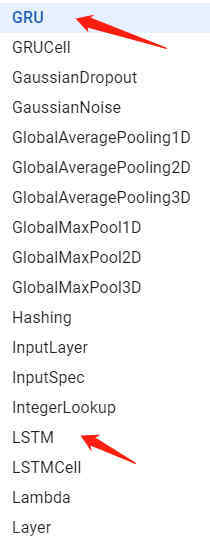self.rnn = nn.LSTM(in_feature, hidden_feature, num_layers) # API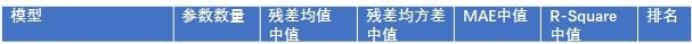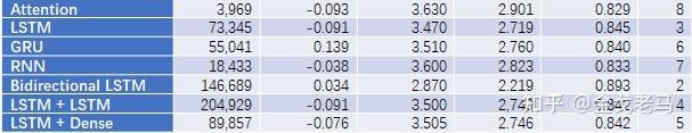## 四、 nn.dynamic_rnn 输出的 final_state.h 和 output[:,-1,:]是否是相同的？

RNN 是这样一个单元：y_t, s_t = f(x_t, s_{t-1}) ，画成图的话，就是这样：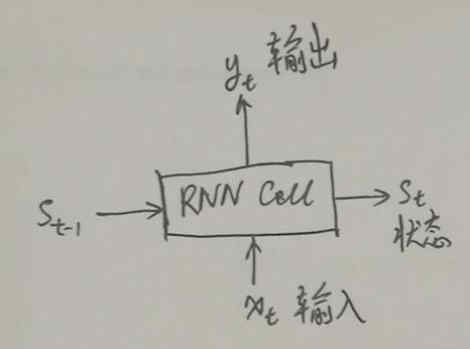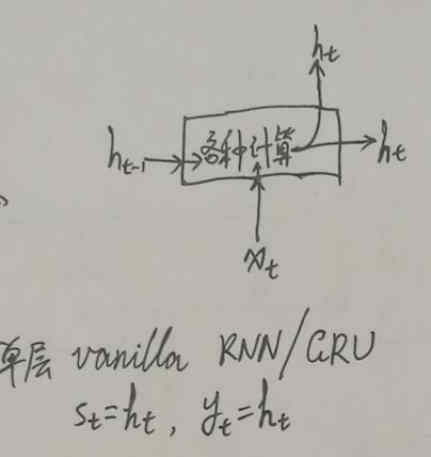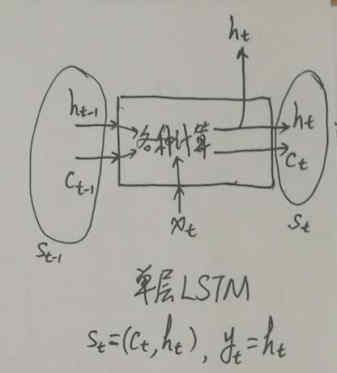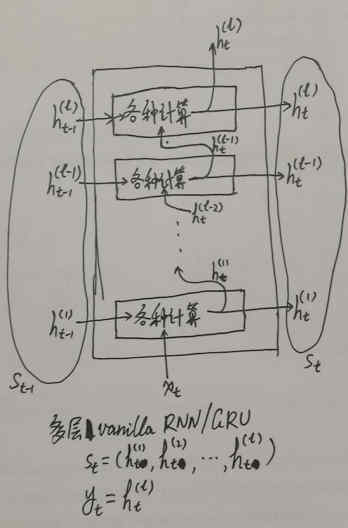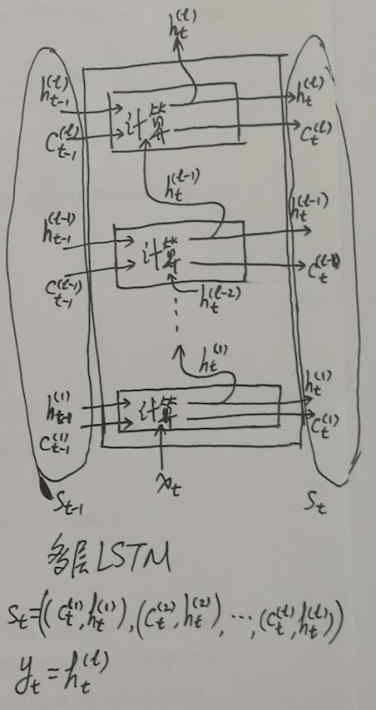output 是 RNN Cell 的 output 组成的列表，假设一共有 T 个时间步，那么 outputs = [y_1, y_2, ..., y_T]，因此 outputs[:,-1,:] = y_T；而 final_state.h 则是最后一步的隐层状态的输出，即 h_T。## 评论